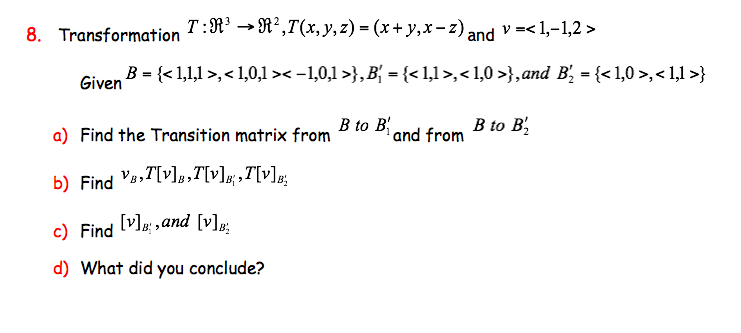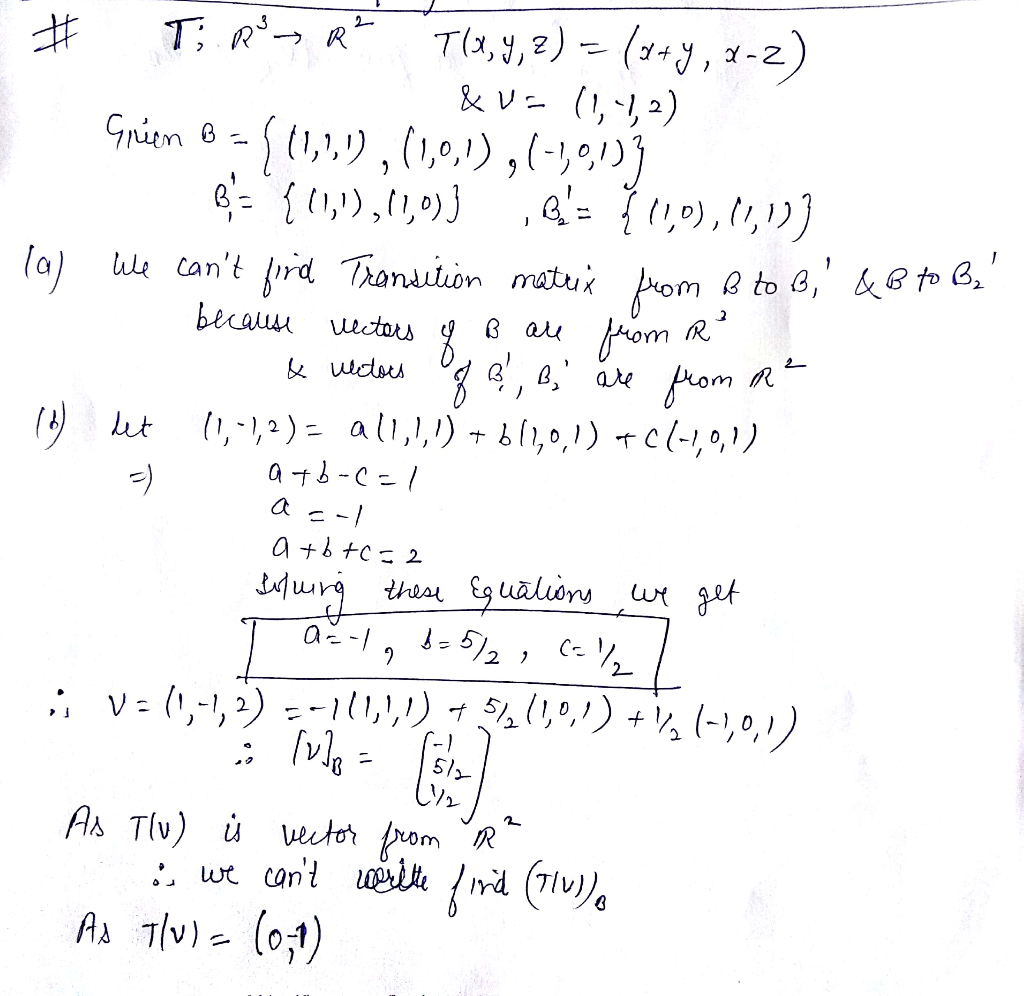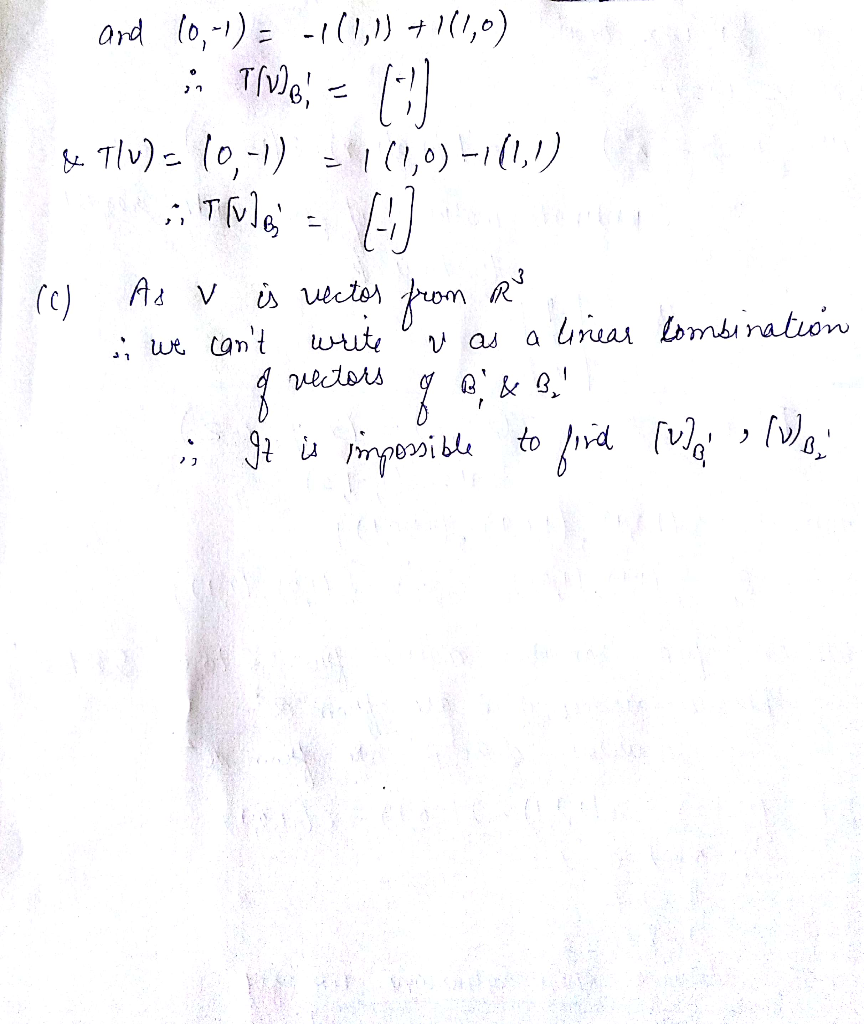# Transformation T:R' -»R',T(x,y, z) = (x+y,x-z)nd v=< 1,-1,2 > 8. ar iven B <1,1,1>,< 1,0,1 >&...Transformation T:R' -»R',T(x,y, z) = (x+y,x-z)nd v= 8. ar iven B ,},B^ = {,},and B, = {,} B to Biand from B to B2 a) Find the Transition matrix from b) Find v],T[v];,7[v] c) Find v,and [v]p d) What did you conclude?##### Add Answer of: Transformation T:R' -»R',T(x,y, z) = (x+y,x-z)nd v=< 1,-1,2 > 8. ar iven B <1,1,1>,< 1,0,1 >&...
More Homework Help Questions Additional questions in this topic.